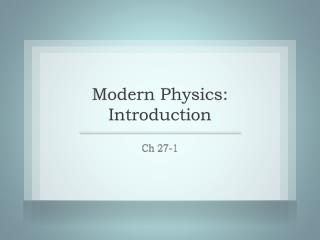# Modern Physics: Introduction - PowerPoint PPT PresentationDownload PresentationModern Physics: Introduction

Modern Physics: Introduction
Download Presentation## Modern Physics: Introduction

- - - - - - - - - - - - - - - - - - - - - - - - - - - E N D - - - - - - - - - - - - - - - - - - - - - - - - - - -
##### Presentation Transcript

1. Modern Physics: Introduction Ch 27-1

2. Greeks first had atomic theory • B.C. • Smallest Unit of “what is” • Atoms explained crystalline shapes • Chemical Reactions always occurred in precise proportions Atoms

3. Avogadro – Equal volumes of gas contain the same number of molecules • Late 1700s – Early 1800s • Mass varies according to the mass of the molecule • Avogadro’s Number – 6.022 x 1023 • Periodic Table • Regular Spacing of Elements Enlightenment

4. Early 1800s • Decomposed substances by passing current through them. • Mass decomposed ∝ time and current • Mass transfer ∝ charge • Charge came only in set values • QUANTIZED! Michael Faraday

5. By the 1880s a number of experiments established the approximate size of the atom • How big is a Copper Atom? • ρcu = 8.96g/cm3 • Atomic mass of Cu = 63.6g/mol • NA = 6.022x1023 atoms/mol • ρ = m/V = mmol/Vmol =mmol/(NA * V1 atom Cu) • V1 atom Cu = mmol/(NA * ρ) = 1.18 x 10-29m3 • If cube V = l*w*h , then 2.3 x 10-10m per side • If sphere V = (4/3)πr3 , then 1.4 x 10-10m radius • 10-10m = 1 Angstrom Size of the Atom

6. 1895 William Roentgen • Cathode Ray Tube • Rays passed through light objects • Exposed film (like light) • Photographs of the shadows of the bones of the hand • Like light, it was not deflected by electrostatic or magnetic fields (NO Charge) • Rays discharge electrified objects X-Rays

7. Roentgen believed X-Rays to be a wave • Diffraction – NO • Reflection – NO • Polarization – NO • Problem – d ~= λ for diffraction to be observed • λ = 0.1nm !!!!! • 1912 first partial diffraction • Roentgen was awarded the 1st Nobel Prize in Physics X-Rays

8. Space between atoms in a crystal is about 0.1nm Crystal Diffraction